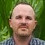## Saturday, January 15, 2011

### The Naive Bayes Classsifier

You may already be familiar with many versions of  Bayes Rule:
p(yi|x) = p(x| yi)p(yi) / p(x)    or

p(y|x) = p(x|y)p(y)/p(x) =  p(x|y)p(y) / ∑i p(x| yi) p(yi) or

p(y = yi |x = xk) = p(x = xk |y = yi)p(y = yi) /∑ j p(x = xk|y = yj)p(y = yj)

Lets assume that y takes on ‘i’ distinct values or classes.  If we know p(x|yi) and p(yi) then we could use Bayes rule to calculate:

p(yi|x) = p(x|yi)p(yi) / p(x)     (1)

However, if x is represented as (x1,…….xk) then there are numerous combinations to consider for each class or value of y. This makes the computation of p(x|yi) computationally demanding. One approach would be to simplify this calculation by making a ‘naïve’ assumption of conditional independence:

Case where k = 2

p(x|y) = p(x1,x2|y) = p(x1|y) p(x2|y) = ∏ p(xk|yi)   (2)  'conditional independence assumption'

the individual x-values are assumed to be independent, conditional on the value of y.

Under the conditional independence assumption, the number of calculations that need to be made for each value of y is decreased dramatically.

Substituting the calculation in (2) into (1) gives us the Naïve Bayes Classifier.

p(yi|x) = ∏ p(xk|yi) p(yi) / p(x)

Like ridge regression, the Naive Bayes Classifier is another example of a biased estimator that can outperform unbiased estimators given its lower variance. From 'Elements of Statistical Learning: data mining, inference and prediction:

" Despite these rather optimistic assumptions, naive Bayes classifiers often outperform far more sophisticated alternatives.....although the individual class density estimates may be biased, this bias might not hurt the posterior probabilities as much, especially near the decision regions. In fact, the problem may be able to withstand considerable bias for the savings in variance such a “naive” assumption earns"

1.Typically p(x|yi) and p(yi) are derived from a Training Set. Then applying these priors, we calculate the Posterior Probabilities using the Bayes Rule.
Since we assume the priors are 'given'.. isnt this infact a Frequentist way of thinking..

Aside question - can I take the posterior probabilities and calculate a GINI/AUC statistic and directly compare it with say a Discriminative method like say Logistic Regression?
I think the answer is it cannot - However, I would like to hear the readers comments.

If not, then what other metrics can we use to compare the performance (mis-classification rates, profit etc.??) Any thoughts?

2.I apologize for taking so long to moderate this and post, I've been out of town at conferences and had spotty internet access. To comment on your questions, I'm not sure. I've only began to think about bayesian methods. (you may check out some of my more recent bayesian tagged posts). They won't answer your questions though, their beyond my current comprehension of bayesian stats. In terms of comparing AUC, if the targets are the same, (such as a binary 0/1) I always thought that the area under the ROC curve was universally comparable across algorithms, say nerual net vs. logistic vs. decision tree etc. I'd be interested in other readers comments on all of the above as well.